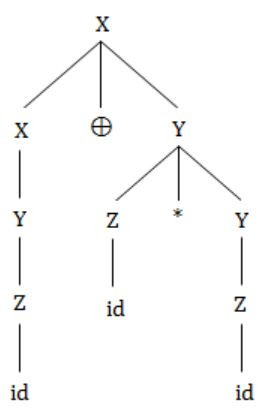## Grammar

 Question 1

In the following grammar

```         X ::= X ⊕ Y/Y
Y ::= Z * Y/Z
Z ::= id  ```

Which of the following is true?

 A ‘⊕’ is left associative while ‘*’ is right associative B Both ‘⊕’ and ‘*’ is left associative C ‘⊕’ is right associative while ‘*’ is left associative D None of the above
Compiler-Design       Grammar       GATE 1997
Question 1 Explanation:⊕ is left associative.
* is right associative.
 Question 2

A grammar that is both left and right recursive for a non-terminal, is

 A Ambiguous B Unambiguous C Information is not sufficient to decide whether it is ambiguous or unambiguous D None of the above
Theory-of-Computation       Grammar       GATE 1999
Question 2 Explanation:
If a grammar is both left and right recursion, then grammar may or may not be ambiguous.
 Question 3

Given the following expression grammar:

```    E → E * F | F + E | F
F → F - F | id  ```

which of the following is true?

 A * has higher precedence than + B - has higher precedence than * C + and – have same precedence D + has higher precedence than *
Compiler-Design       Grammar       GATE 2000
Question 3 Explanation:
The operator which is in low level that can have high preference.
Order of precedence is *, +, -.
Here * and + have equal preference, '-' can have higher precedence than + and *.
 Question 4

Which of the following suffices to convert an arbitrary CFG to an LL(1) grammar?

 A Removing left recursion alone B Factoring the grammar alone C Removing left recursion and factoring the grammar D None of the above
Compiler-Design       Grammar       GATE 2003
Question 4 Explanation:
Left recursion removing (or) factoring the given grammar are not sufficient to convert an arbitrary CFG to an LL(1) grammar.
To convert an arbitrary CFG to an LL(1) grammar we need to remove the left recursion and as well as left factoring without that we cannot convert.
There are 4 questions to complete.

Register Now